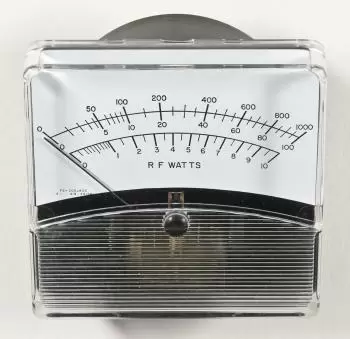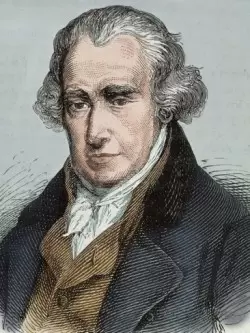# KilowattOne kilowatt (kw) is a power unit in current use, equivalent to 1000 watts (w).

One watt (w) is a power unit of the international system equivalent to one joule per second.

If we express the watt in units used in electricity, we can say that a watt is the electric power produced by a potential difference of 1 volt and an electric current of 1 ampere (1 volt ampere).

Frequently the watt hour (Wh) is also spoken as an energy unit. The watt hour is a practical unit of energy equivalent to the energy produced by a power of one watt for one hour.

Sometimes the kilowatt is confused with other related units of measure but representing different values.

### Watt and Watt-hour

Power and energy are easy to confuse. It can be said that power is the rhythm of consumption (or generation) of energy. One watt is one joule per second. For example, if a 100 W bulb stays lit for one hour, the energy consumed is 100 watt-hours (W · h) or 0.1 kilowatt-hours (kW · h) or (60 × 60 × 100) 360,000 joules (J). This would be the same amount of energy that would make a 40 W bulb shine for 2.5 hours. The capacity of a power plant is measured in watts, but the energy generated annually is measured in watt-hours.

This last unit is not usually used, normally it is passed directly to the kilowatt-hour, or megawatt-hour. The kilowatt-hour (kWh) is not a unit of power, it is a unit of energy. It is common to confuse them due to the tendency to shorten the term energy by using kilowatt instead of kilowatt-hour.

### Watt-hour and Watt per Hour

Annual consumption data and capacity. Another source of confusion can be caused by the use of incorrect terminology when mentioning the energy in kWh, which can be confused if it is read as if it were kilowatt hour or kW / h. This type of unit is related to the generation of energy and can be interesting to express the characteristics of a power plant.

Units of the previous type, such as Watt per hour (W / h) reflect the capacity to change the power per hour. The watts per hour (W / h) are useful to characterize the speed of increase of power in the generation plants. For example, a power plant that reaches a power of 1 MW from zero to 15 minutes has a rate or speed of increase of its power of 4 MW / h. Hydroelectric plants have a very high rate of power increase, which makes them very capable of facing maximum loads and emergency situations.

The majority of energy production or consumption in a period expressed in terawatt hours · hour consumed or produced. The period that is used is normally a calendar year or a financial year. A terawatt-hour equals a continuous consumption (or production) of energy of approximately 114 megawatts over a period of one year.

Sometimes a balance is made of the energy consumed in a year that would represent the installed power making more visible transformations for the receivers of the reports, for example, the continuous consumption of 1 kW during a year would result in a need in terms of energy of about 8760 kW · h / year. Sometimes we talk about watts · year at conferences in the field of global warming and energy consumption.

### Difference Between W and W 'In many physics books the W notation to denote the work (from the English word work) is included and must be distinguished from the units in watts (work / time). Normally, the books, write the work with the letter W in italics or with a similar drawing to the raised hand.

## Origin of the Name Watt (w)

The watt is named after the Scottish scientist James Watt for his contributions to the development of the steam engine.

The unit of measurement was recognized by the Second Congress of the British Association for the Advancement of Science in 1882, coinciding with the start of commercial production of electricity from water and steam.

In 1960 the 11th General Conference on Weights and Measures adopted this unit of measure for the measurement of power in the International System of Units (SI).

Author:

Published: May 13, 2015
Last review: May 28, 2020